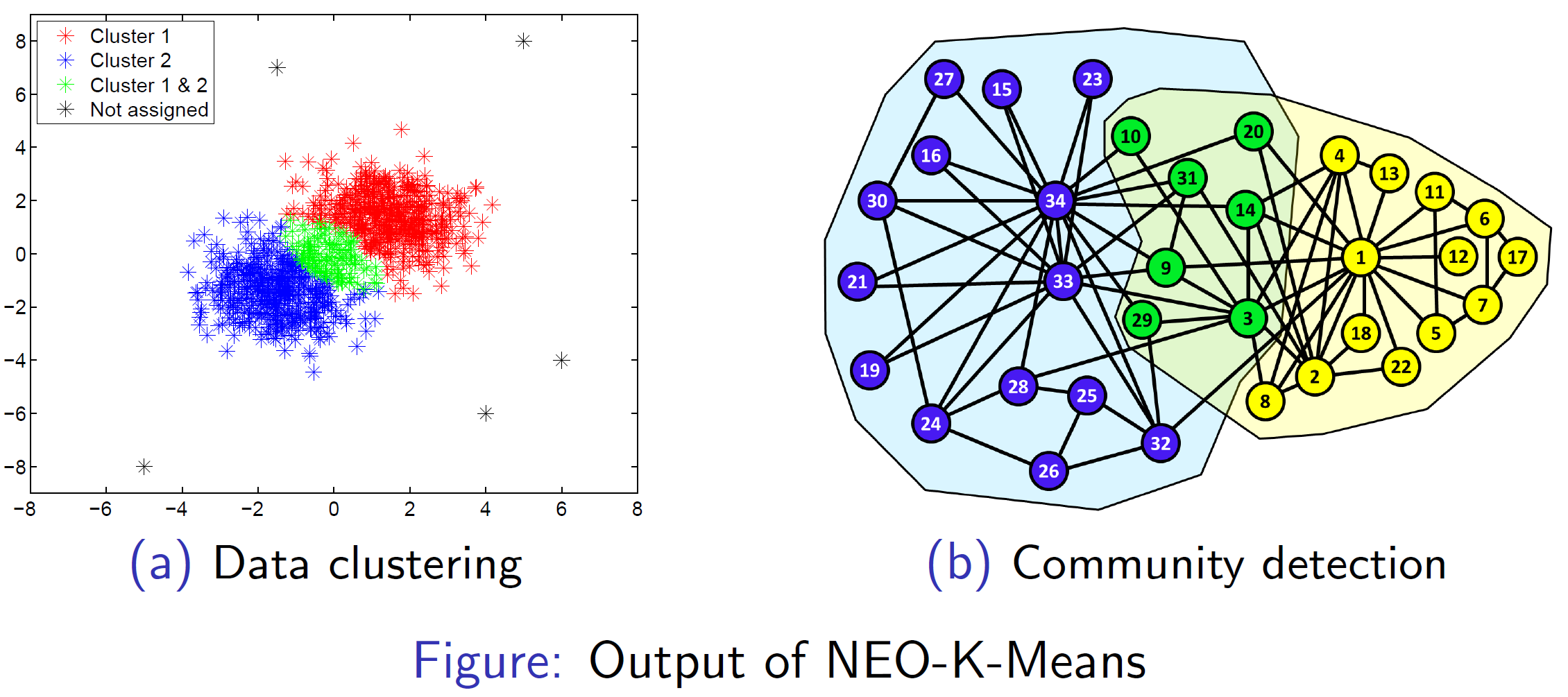# NEO-K-Means

• NEO-K-Means (Non-exhaustive, Overlapping K-Means)
We propose a simple and intuitive objective function that captures the issues of overlap and non-exhaustiveness in a unified manner. Our objective function can be viewed as a reformulation of the traditional k-means objective, with easy-to-understand parameters that capture the degrees of overlap and non-exhaustiveness. To optimize the objective, we propose a simple iterative algorithm which we call NEO-K-Means (Non-Exhaustive Overlapping K-Means). Our NEO-K-Means algorithm can be used for both data clustering and graph clustering.

The NEO-K-Means algorithm for data clustering is written in MATLAB and the algorithm for graph clustering is written in a mixture of C++ and MATLAB.

### -Data Clustering

A sample dataset (synth2.mat) and a script file (run_synth2.m) are included.

Please run the script to test the code and visualize the clustering result.

### -Graph Clustering

In the command line, please type:

make clean

make

And then, you can run the code using the following command:

./neo -a alpha_value -b beta_value -s sigma_value input (Please set the sigma_value to be zero.)

For example, you can just type sh run_neo.sh to see how it works on the karate club network.

In the output file, each line corresponds to the membership of the node. For example, if the first line contains 0 and 1, it means that the first node belongs to cluster 0 and cluster 1.

You can also find the MATLAB interface within ‘matlab’ folder. Please run ‘test.m’ to test the code on the karate club network. You might want to execute MATLAB using the following command: LD_PRELOAD=”/usr/lib/x86_64-linux-gnu/libstdc++.so.6″ matlab

### Citation

This software is released under the GNU Public License (GPL) but please acknowledge its use with a citation to at least one of the following publications: